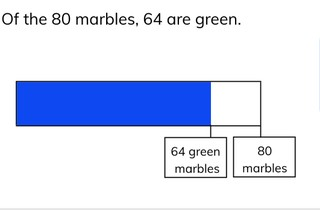Determining fractions

# Determining fractions

I can determine which fraction matches the given information.8,000 schools use Gynzy92,000 teachers use Gynzy1,600,000 students use Gynzy

## General

Students learn to determine which fraction is represented by a given set of numbers or information given.

## Common core standard(s)

CCSS.MATH.CONTENT.3.NF.A.3.B
CCSS.MATH.CONTENT.4.NF.A.1

## Relevance

It is important to learn this so that you can quickly determine how much of a total you have and express that as a fraction.

## Introduction

Students name the fractions shown by the fraction bar on the interactive whiteboard.

## Development

Refresh with students that fractions are built up with a numerator and denominator. Explain the difference between a base fraction and a regular fraction. Repeat the steps that students need to take to simplify a fraction. Emphasize that you try to take the largest number possible to divide the fraction to try to simplify the fraction to as small a fraction (numerically) as possible. Show the problem with the marbles and the fraction bar. Explain that you first need to determine which fraction is shown with the information given. That is 64//80. Now you have to divide the numerator and the denominator by the largest possible number. They are both divisible by 16, so you divide 80 ÷ 16 = 5 and 64 ÷ 16 = 4. The simplified fraction is 4//6. Ask students to solve the two problems given to check that they can determine the fraction from the fraction bar as visual support. Next explain to students how to to determine a fraction from a visual representation. Emphasize that you first count the total number of boxes shown, and then that you count the number that you want to know (all of one color for example). The fraction shown in this image is 6//9. Again, remind students that they have to simplify. Divide the fraction by 3 to simplify to 2//3. Check with the given exercises that students are able to determine the fraction when only a visual is given. Next show students how to understand "8 of the 64" as the fraction 8//64. Simplify the fraction by dividing by 8 and you get 1//8. Check that students understand by having them solve the next two problems on the interactive whiteboard. Finally explain the steps of the story problem. Make sure that students carefully determine which numbers are needed to solve the story problem and simplify the fraction. Ask students to select the correct multiple choice answer by the next two story problems.

Check that students are able to determine fractions by asking the following questions:
- How do you determine which fraction matches the information?
- How do you simplify a fraction?

## Guided practice

Students practice determining fractions. They are given problems with visual support from a fraction bar, then with only the numbers given, and then students get story problems.

## Closing

Discuss with students why it is important to be able to determine fractions of given quantities. To close ask students to form pairs and correct the mistake. In the first problem the answer is not incorrect, but not simplified enough. In the second problem, the fraction given is for a different color.

## Teaching tips

Students who have difficulty determining fractions can be supported by figuring out what the fraction is using a fraction circle or fraction bar.

### The online teaching platform for interactive whiteboards and displays in schools

• Save time building lessons

• Manage the classroom more efficiently

• Increase student engagement